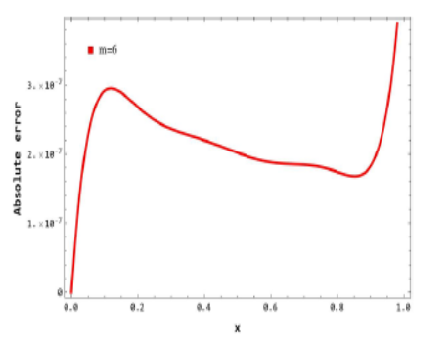• P-ISSN 0974-6846 E-ISSN 0974-5645# Indian Journal of Science and Technology

## Article• VIEWS 266
• PDF 71

Indian Journal of Science and Technology

Year: 2023, Volume: 16, Issue: 15, Pages: 1159-1167

Original Article

## Numerical Solution of Non-linear Integro-differential Equations using Operational Matrix based on the Hosoya Polynomial of a Path Graph

Received Date:22 December 2020, Accepted Date:30 March 2023, Published Date:21 April 2023

## Abstract

Objectives: Introduction to new numerical techniques to solve differential, difference, and integro-differential equations (IDEs) are always remaining the thrust area of research for many scientists over the centuries. The prime objective of this work is to contribute a new numerical technique to solve IDEs. Method: To address non-linear integro-differential equations, we computed an operational matrix of derivatives based on the Hosoya polynomial of the path graph in this work. Findings: Using the derived operational matrix, we have solved both Volterra and Fredholm integrodifferential equations. Taking suitable examples accuracy of the projected method is demonstrated in this paper in terms of a graphical representation of the absolute error. The results of the examples reveal that the projected method is a suitable method to solve IDEs. Novelty: The application of the Hosoya polynomial of path graph to solve integro-differential equations is a novel approach in the field of numerical analysis. Keywords: Volterra Integrodifferential equations; Fredhlom Integrodifferential equations; Graph theorypolynomials

## References

1. Jalilian R, Tahernezhad T. Exponential spline method for approximation solution of Fredholm integro-differential equation. International Journal of Computer Mathematics. 2020;97(4):791–801. Available from: https://doi.org/10.1080/00207160.2019.1586891
2. Hamoud AA, Mohammed NM, Ghadle KP, Dhondge SL. Solving Integro differential equation by using numerical techniques. International Journal of Applied Engineering and Research. 2019;14(14):3219–3225. Available from: https://www.ripublication.com/ijaer19/ijaerv14n14_13.pdf
3. Ji T, Hou J, Yang C. The operational matrix of Chebyshev polynomials for solving pantograph-type Volterra integro-differential equations. Advances in Continuous and Discrete Models. 2022;2022(1):57. Available from: https://doi.org/10.1186/s13662-022-03729-1
4. Singh AK, Madhulika M, Arora R. Numerical solution of integro-differential equations by using Euler's operational matrix of integration. Science and Aerospace (MESA). 2021;12(2):1–6.
5. Raslan KR, Ali KK, Mohamed EMH, Mohamed EMH, salam MAAE. An Operational Matrix Technique Based on Chebyshev Polynomials for Solving Mixed Volterra-Fredholm Delay Integro-Differential Equations of Variable-Order. Journal of Function Spaces. 2022;2022. Available from: https://doi.org/10.1155/2022/6203440
6. Sadri K, Hosseini K, Mirzazadeh M, Ahmadian A, Salahshour S, Singh J. Bivariate Jacobi polynomials for solving Volterra partial integro‐differential equations with the weakly singular kernel. Mathematical Methods in the Applied Sciences. 2021;p. 1–17. Available from: https://doi.org/10.1002/mma.7662
7. Hamoud AA, Ghadle KP. Homotopy Analysis Method for the First Order Fuzzy Volterra-Fredholm Integro-differential Equations. Indonesian Journal of Electrical Engineering and Computer Science. 2018;11(3):857–867. Available from: http://doi.org/10.11591/ijeecs.v11.i3.pp857-867
8. Hamoud AA, Dawood LA, Ghadle KP, Atshan SM. Usage of the modified variational iteration technique for solving Fredholm integro-differential equations. International Journal of Mechanical and Production Engineering Research and Development. 2019;9(2):895–902. Available from: http://dx.doi.org/10.12732/ijam.v33i2.5
9. Issa MSB, Hamoud AA, Ghadle KP. Numerical solutions of fuzzy integro-differential equations of the second kind. Journal of Mathematics and Computer Science. 2021;23(1):67–74. Available from: http://dx.doi.org/10.22436/jmcs.023.01.07Article Metrics